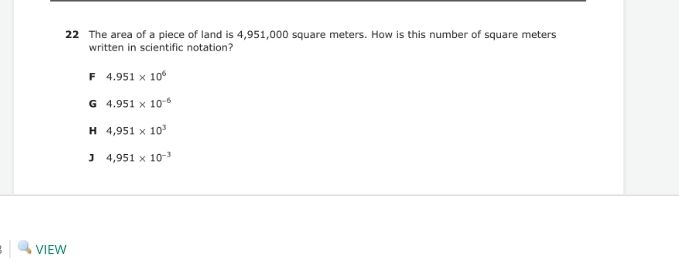### ¿Todavía tienes preguntas de matemáticas?

Pregunte a nuestros tutores expertos
Algebra
Pregunta$$22$$ The area of a piece of land is $$4,951,000$$ square meters. How is this number of square meters Written in scientific notation?

F $$4.951 \times 10 ^ { 6 }$$

$$4.951 \times 10 ^ { - 6 }$$

$$4,951 \times 10 ^ { 3 }$$

$$4,951 \times 10 ^ { - 3 }$$